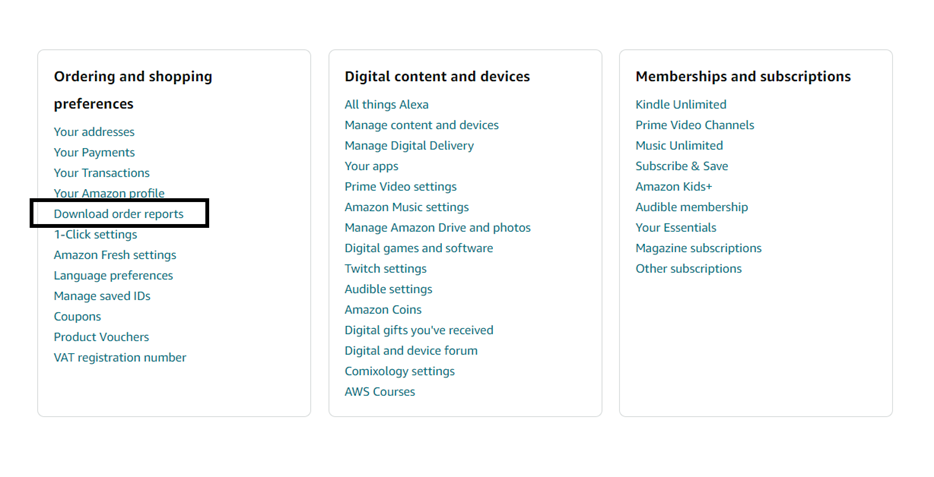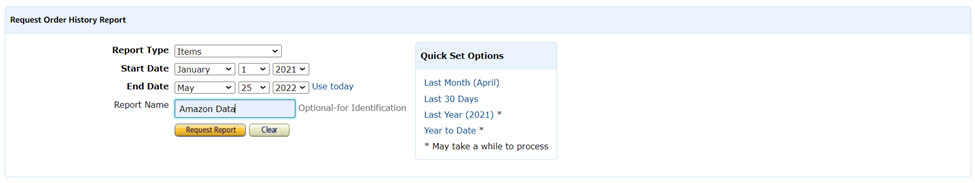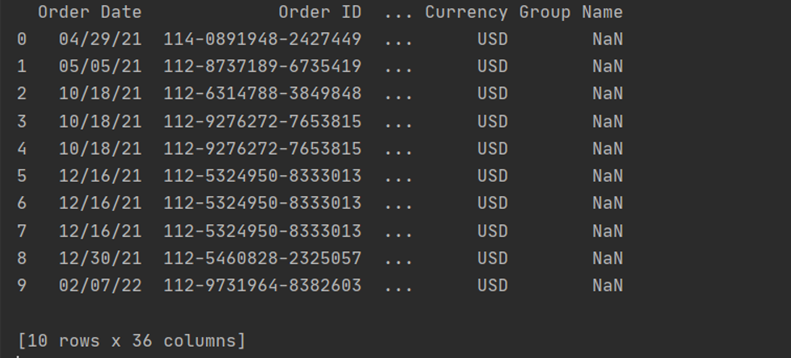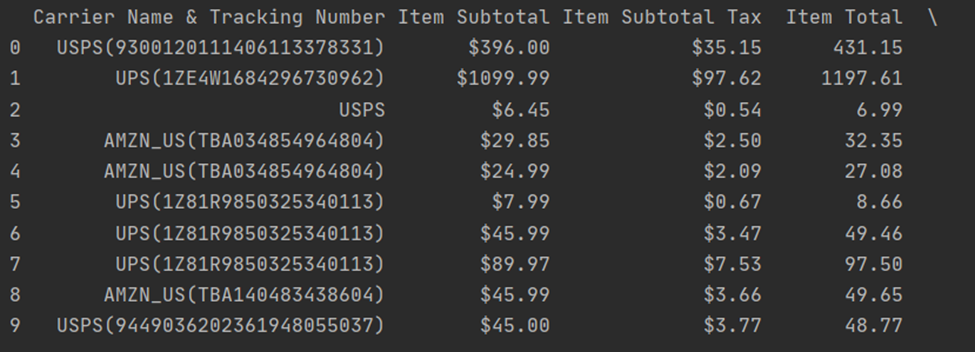# Analyze Your Amazon Data with Python

Mr. Unity Buddy

We’re Earthly. We simplify and speed up software building with containerization. Working with Python and Pandas for data analysis? Earthly can be a game-changer for your CI tests! Give it a try.

How much have you spent on Amazon? Well, that’s a kind of interesting question to find an answer to. And it’s the type of question I like to answer using Python.

With Python, Data analysis is just a 10-minute job. So in this article, we’re going to analyze your Amazon data with a few lines of code. By the end of the article, we will have:

• Figured out how much money we’ve spent on Amazon
• Found the most expensive and cheapest things we’ve ordered.
• Found the average of our expenses on Amazon
• Found out how much we have spent on Tax
• And we’ll also visualize our spending habits day by day in a graph.

## Getting Started

Before we get started, we need to set up a few things. We’ll need:

• A Python IDE and Python 3.x
• Pandas Library (It is a built-in library but in case it is not right there, install it through `pip install pandas`)
• Matpotlib installed (to install, use `pip install matpotlib`)

Step 2: Click on Your Account > AccountStep 3: In the Order and Shopping Preferences section, select “Download order reports”Step 4: Customize the data according to your need and click on “Request Report”.Congrats! You’ve your order history stored in a CSV file. Now we can move to code.

## Find out How Much Have You Spent on Amazon

Python is an excellent programming language for data analysis. And a library that makes Python perfect for data analysis is Pandas. However, before starting to use it, it would be great to know what exactly it is.

### What Is Pandas?

Pandas is a Python library used for working with data sets. It is an open-source, free-to-use library that saved the jobs of millions of data scientists and other people!

Pandas have so many uses that it is probably easier to list the things it can’t do. Through pandas, you get acquainted with your data by cleaning, transforming, and analyzing it.

It has some built-in functions which help us to analyze data files easily. Some of the commonly used general commands are below:

`pd.read_filetype()` - To open a file

`pd.DataFrame()`- To Convert a Python object (Lists, Dicts, etc) to a DataFrame.

`Df.shape()` - To show the number of columns and rows

`df.head()`- Show the first 5 rows of the data frame

`Df.info()` - To get information, like Data type, index, memory, etc

`df.describe()` - To get the statics summary for each numerical column (Mean, Maximum, Minimum, etc)

### Getting Data Into Pandas

First of all, let’s open and read the CSV file from pandas. That’s just 4 lines of code:

``````import pandas as pd

pd.set_option('display.max_columns', 5) # display first 5 columns only
print(df)``````

The output is 5 columns with a row for each record in the CSV file (We’re using a small data frame for the tutorial so your data frame can be a lot bigger!):We can customize the number of rows and columns anytime.

### Cleaning Data

In Data analysis, Cleaning data is essential. Let’s start by dealing with those NaN values. We need to do some math with our data, but 1 + NaN is a somewhat challenging math problem to solve. After all, NaN is not a number or an integer. In simple words, NaN represents the absence of information. So we have to fill in those NaN values using a handy function—`df.fillna`. Using this function, we can automatically replace every NaN value with 0.

But we need to be careful here. Since we don’t want to replace those values temporarily, we should replace them and store them in our new data frame. We will use `df = df.fillna(0)` to replace the values and re-define our df variable to that new data frame.

We can modify our code like this:

``````import pandas as pd

pd.set_option('display.max_columns', 36) # display all the columns
df = df.fillna(0)
print(df)``````

If you take a look at the previous output and the current output you’ll see that null values have been replaced by 0. But we have one more problem with the Prices. The value in the prices column is stored in String format and also the string contains a dollar sign. . So before we start the calculation, we have to remove the dollar signs and convert string values to floats. ( Floats are a python data type used to represent real numbers. They are written with a decimal point dividing the integer and fractional parts).

As I said before, Pandas is born to make our data analyst’s life easier. Solving this problem is so easy with Pandas.

We can use `Series.str.replace()` to replace one character or a set of characters with another in any column. (a column is called a series in Pandas) of a data frame. We can use the syntax`df["Item Total"].str.replace('\$', "")` to tell Pandas to replace the first set of “\$” in the Item Subtotal column with ""(nothing).

Again, we don’t want to replace dollar signs temporarily, we should modify the data frame first and then point the df variable to the new version. So the improved code looks like this:

``````import pandas as pd

pd.set_option('display.max_columns', 36) # display all the columns
df = df.fillna(0)
df["Item Total"] = df["Item Total"].str.replace('\$','')
print(df)``````

Output:As you see, there’s no dollar sign in “Item Total” column. Cool, we can jump into the next problem. What we want now is to convert string values in the Item Subtotal column to float.

Pandas has a method for this purpose, too. `df.astype()` allows us to convert entries into another datatype. We simply have to add `.astype(float)` to the code we have written.

``````import pandas as pd

pd.set_option('display.max_columns', 36)
df = df.fillna(0)
df["Item Total"] = df["Item Total"].str.replace('\$','').astype(float)
print(df)``````

You can’t see the values are either strings or floats. We will get to see the result in our next step.

### Calculating the Total Amount of Money Spent on Amazon

Pandas offer built-in calculation methods that we can use to play with these columns. The .sum() method is used to calculate the total of every number in a column. That will give us the total amount of money we’ve spent on Amazon. Excited? Run this code.

``````import pandas as pd

pd.set_option('display.max_columns', 36)
df = df.fillna(0)
df["Item Total"] = df["Item Total"].str.replace('\$','').astype(float)
print(df)
print(df["Item Subtotal"].sum())``````

My result for this data frame was:

``1949.2199999999998``

It means I’ve spent \$1942.21 on Amazon. That surprised me!. Did you get surprised too?

## Finding the Biggest Purchase, Average, etc

Since we have cleaned our data frame now it’s easy to calculate other things.

First, let’s find the Biggest purchase you’ve made on Amazon. For this, we can use a simple, built-in method, `df.max()`:

``````import pandas as pd

df = df.fillna(0)
df["Item Total"] = df["Item Total"].str.replace('\$','').astype(float)

print(df["Item Total"].max())``````

The result was:

``1197.61``

If you want to find out what the item was, you can look at the CSV file.

What is my small purchase if my biggest purchase was \$1197? Finding out the smallest order is too easy with df.min() function.

We just remove the .max() and place .min()

``````import pandas as pd

df = df.fillna(0)
df["Item Total"] = df["Item Total"].str.replace('\$','').astype(float)

print(df["Item Total"].min())``````

And the output was:

``6.99``

Cool, my lowest-priced purchase was a battery worth \$6.99! What was yours?

Next, let’s find out what the average spend per order on Amazon is. Doing so is easy with the help of df.mean() function.

Here’s the code we can use:

``````import pandas as pd

df = df.fillna(0)
df["Item Total"] = df["Item Total"].str.replace('\$','').astype(float)
print(df["Item Total"].mean())``````

Result:

``194.92199999999997``

It looks like my average spend on Amazon is \$194.92. Not bad!

But looking at the mean/average can sometimes be misleading, it’s nice to check out the median as well. We can use .median() to do that.

Let’s change the code to:

``````df = pd.read_csv('C:\\Users\\nethm\\Downloads\\AmazonData.csv')
df = df.fillna(0)
df["Item Total"] = df["Item Total"].str.replace('\$','').astype(float)

print(df["Item Total"].median())``````

Result:

``134.115``

As you see, the results aren’t the same. But we don’t have to worry about it, we can decide that our average spend is from \$134 - \$194.

## Find out How Much You’ve Spent on Tax

In all the above, we’ve played with only one column. What if we want to find the amount of Tax we’ve spent in another column? That’s also simple, we just have to re-do the steps.

First of all, we should remove the dollar signs in the “Item Subtotal Tax” column and convert the string values to floats.To do this, we can improve our code like this:

``````import pandas as pd

pd.set_option('display.max_columns', 36) df = df.fillna(0)
df["Item Subtotal Tax"] = df["Item Subtotal Tax"].str.replace('\$','').astype(float)
print(df)``````

Now we can see that the dollar signs in front of the “Item Subtotal Tax” column have been removed.Now we can easily use sum() function on that too.

``````import pandas as pd

pd.set_option('display.max_columns', 36)
df = df.fillna(0)
df["Item Subtotal Tax"] = df["Item Subtotal Tax"].str.replace('\$','').astype(float)
print(df)
print(df["Item Subtotal Tax"].sum())``````

Output:

``157.0``

I have paid \$157 on Tax. The tax rate on different items isn’t the same, and some items don’t have tax. But if you want to find the overall tax rate, we have to divide some of the Item Subtotal Tax column from the sum of the Item Total column like this:

``````import pandas as pd

pd.set_option('display.max_columns', 36)
df = df.fillna(0)
df["Item Subtotal Tax"] = df["Item Subtotal Tax"].str.replace('\$','').astype(float)
df["Item Total"] = df["Item Total"].str.replace('\$','').astype(float)
print(df)
print(df["Item Subtotal Tax"].sum() / df["Item Total"].sum())``````

Result:

``0.08054503852823182``

That means the tax rate was nearly 8%. Well, that’s a little bit high but fine.

## Analyze Your Amazon Spend over Time

Do you want to find out which days you have spent most on Amazon? Alright, we can do it! Moreover, we can make a bar chart with matpotlib too.

Before working with dates, we have to convert them to datetime data types so Python can recognize them as dates. Luckily, Pandas has a built-in method— `pd.to_datetime().`

``````import pandas as pd

pd.set_option('display.max_columns', 36)
df = df.fillna(0)
df['Order Date'] = pd.to_datetime(df['Order Date']).dt.date #converting to date format, without time

print(df)``````

Great, now we can see that the “Order Date” column has been changed to the default date-time format.It’s time to move to analyze step. Which dates have we spent most of the money on Amazon? Let’s graph it with “Matpotlib” “Matpotlib” makes it easier to make bar graphs using data. Let’s move!

The basic syntax of making a bar chart in pandas Matpotlib is like this:

``df.plot.bar(x,y, rot, color)``

In this, x means the x-axis, and “y” represents the y-axis.`rot` keyword is used to rotate text on the x-axis and the color keyword is used to change the color of the bar chart.

So we can write our code according to that syntax, like this:

``````import matplotlib.pyplot as plt
import pandas as pd

df = df.fillna(0)
df['Order Date'] = pd.to_datetime(df['Order Date']).dt.date

df["Item Total"] = df["Item Total"].str.replace('\$','').astype(float)
bar = df.plot.bar(x='Order Date', y='Item Total', rot=0, color="#36b6fa")

plt.show()``````

Run the code, and there will be an awesome bar chart like the below:But if your CSV file contains a lot of rows, the bar chart would be unclear and very small in size. To zoom in, we can use the fig size keyword. You can change the code like below:

``bar = df.plot.bar(x='Order Date', y='Item Total', rot=30, color="#36b6fa", figsize=(20, 30))``

Awesome, now we have a beautiful bar chart representing how much we’ve spent on different dates!

## Conclusion

In this tutorial, we explored data analysis using Python libraries like Pandas and Matplotlib. Key lessons include:

• Pandas can greatly simplify data analysis with its numerous built-in functionalities.
• Pre-processing and cleaning data is a crucial step before performing any analysis.
• Visualizations using Matplotlib can help to interpret data more effectively.

As you continue to explore Python and its powerful libraries, you might also be interested in automating your build processes. If so, give Earthly a try. It can help streamline your development workflow, especially if you’re working with complex projects.

Earthly makes builds simple
Fast, repeatable builds with an instantly familiar syntax – like Dockerfile and Makefile had a baby.

Mr. Unity Buddy

Technical writer, Content creator, Game Designer, and a Mad Developer. Visit unitybuddy.com for more!

Published: